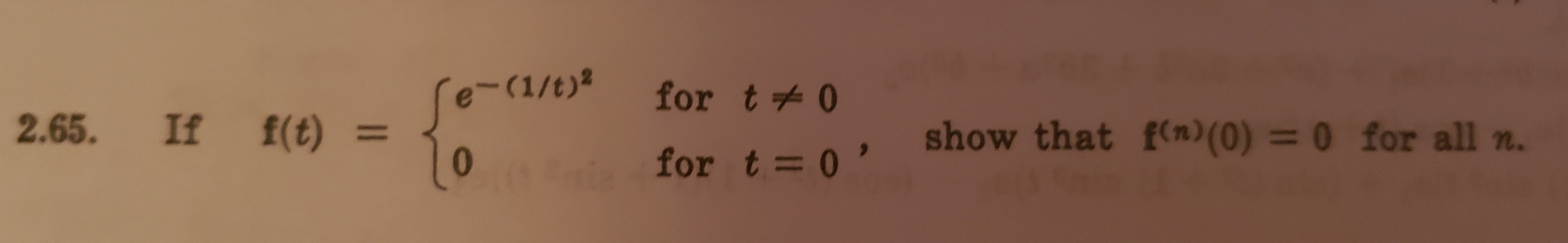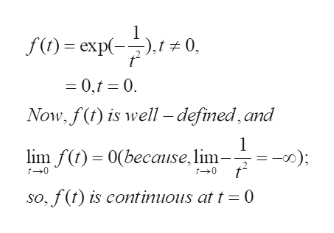-(1/t)2for t02.65. If f(t) =show that f(n) (0) = 0 for all nfor t= 0'

Questionhelp_outlineImage Transcriptionclose-(1/t)2 for t0 2.65. If f(t) = show that f(n) (0) = 0 for all n for t= 0' fullscreen
Step 1

To show that the function f(x) , as defined , has all its derivatives 0 at x =0

Step 2

To start with, f(t) is well defined and continuous...help_outlineImage Transcriptionclosef()exp( .t 0 = 0,t 0 Now. f() is well -defimed,and 1 ) (because, lim- lim f 0 so, f(t) is continuous at t = (0 fullscreen

Want to see the full answer?

See Solution

Want to see this answer and more?

Our solutions are written by experts, many with advanced degrees, and available 24/7

See Solution
Tagged in

Math